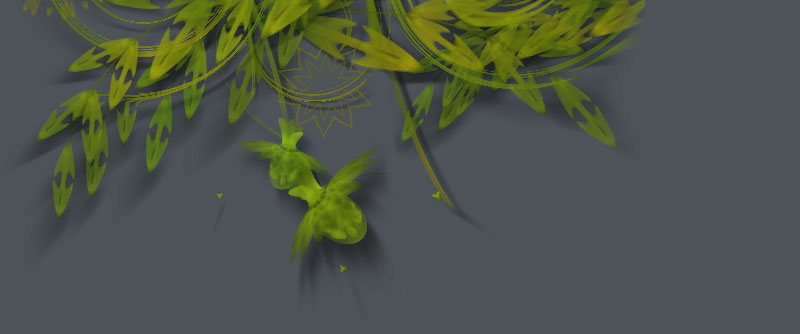### Obstacle avoidance```# --- GEOMETRY --------------------------------------------------------------

from math import degrees, atan2
from math import sqrt, pow
from math import radians, sin, cos

def angle(x0, y0, x1, y1):
""" Returns the angle between two points.
"""
return degrees( atan2(y1-y0, x1-x0) )

def distance(x0, y0, x1, y1):
""" Returns the distance between two points.
"""
return sqrt(pow(x1-x0, 2) + pow(y1-y0, 2))

def coordinates(x0, y0, distance, angle):
""" Returns the coordinates of given distance and angle from a point.
"""
return (x0 + cos(radians(angle)) * distance,

# --- WORLD -----------------------------------------------------------------

class World:
def __init__(self):
self.obstacles = []

# --- OBSTACLE --------------------------------------------------------------

class Obstacle:
self.x = x
self.y = y

# --- CREATURE --------------------------------------------------------------

class Creature:

def __init__(self, world, x, y, speed=1.0, size=4):

self.x = x
self.y = y
self.speed = speed
self.size = size

self.world = world
self.feeler_length = 25

self._vx = 0
self._vy = 0

""" Returns the creature's heading as angle in degrees.
"""

return angle(self.x, self.y, self.x+self._vx, self.y+self._vy)

def avoid_obstacles(self, m=0.4, collision=4):

# Find out where the creature is going.

for obstacle in self.world.obstacles:

# Calculate the distance between the creature and the obstacle.
d = distance(self.x, self.y, obstacle.x, obstacle.y)

# Respond faster if the creature is very close to an obstacle.
if d - obstacle.radius < 4: m *= 10

# Check if the tip of the feeler falls inside the obstacle.
# This is never true if the feeler length
# is smaller than the distance to the obstable.
if d - obstacle.radius <= self.feeler_length:
tip_x, tip_y = coordinates(self.x, self.y, d, a)
if distance(obstacle.x, obstacle.y,

# Nudge the creature away from the obstacle.
m *= self.speed

if tip_x < obstacle.x: self._vx -= random(m)
if tip_y < obstacle.y: self._vy -= random(m)
if tip_x > obstacle.x: self._vx += random(m)
if tip_y > obstacle.y: self._vy += random(m)

if d - obstacle.radius < 4: return

def roam(self):

With its feeler it will scan for obstacles and steer away.
"""

self.avoid_obstacles()

v = self.speed
self._vx += random(-v, v)
self._vy += random(-v, v)
self._vx = max(-v, min(self._vx, v))
self._vy = max(-v, min(self._vy, v))

self.x += self._vx
self.y += self._vy

# ---------------------------------------------------------------------------

size(550, 200)

# Create a world with obstacles at random positions.
world = World()
for i in range(15):
obstacle = Obstacle(random(WIDTH), random(HEIGHT), random(10, 30))
world.obstacles.append(obstacle)

# Create a number of ants.
ants = []
for i in range(4):
ant = Creature(world, 225, 100, speed=2.0)
ants.append(ant)

speed(30)
def draw():

background(0.2)

# Draw all the obstacles in the world.
fill(0.5, 0.5)
for obstacle in world.obstacles:

# Draw each ant and its feeler.
stroke(1)
fill(1, 0.5)
for ant in ants:
push()
transform(CORNER)
translate(ant.x, ant.y)